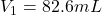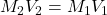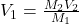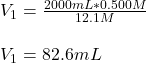## If hydrochloric acid is obtained commercially at a concentration of 12.1M, how many milliliters of 12.1M HCl(aq) must be used to prepare 2.0

Question

If hydrochloric acid is obtained commercially at a concentration of 12.1M, how many milliliters of 12.1M HCl(aq) must be used to prepare 2.00x103mL of 0.500M HCL(aq)?

in progress 0
2 months 2021-07-30T01:57:29+00:00 1 Answers 2 views 0Explanation:

Hello there!

In this case, according to this question, we will need to deal with this dilution problem, because it is asking for the volume of a 12.1-M stock solution of HCl. In such a way, we can use the following equation, under the assumption of no change in the number of moles in the solution:Thus, we solve for the initial volume, V1, as shown below:And plug in the initial concentration and final concentration and volume to obtain:Regards!